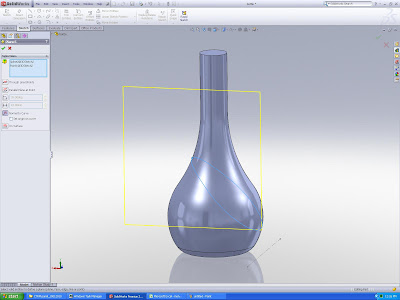Select PageHi all, here is the wine bottle that I share with you previously. Wish to know how to create a ring surrounding the bottle? Actually is very simple, you just need to do the follwoing steps:1. Create an angle plane that intersect with the bottle body. Here, I will refer the angle plane to the current TOP Plane. Open a sketch on the TOP plane and draw a construction line as shown in the picture.2. Then, when using the Reference geometry > Plane funtion, select the construction line to define the plane position and also the TOP plane as the reference to measure the angle.3. With the angle plane, now we can create the path of the ring by using the “Intersection Curve” function. Basically, intersection is a function under “Tools> Sketch Tools> Intersection Curve which enable the user to define 3D curve to represent the intersection between 2 surfaces/solidbodies/planes.4. Now, we can prepare the plane for the sweep profile. You just need to select the 3D curve and pick a point in order to create a plane normal to the curve. Then just draw a circle on the plane as the sweep profile.5. Create the ring using seep function, define the 3D curve as sweep path and the circle as sweep profile.

6. After you get the 1st ring, just use the circular pattern function to get the 2nd and 3rd copy and play around with the angle of rotation then you will get the unexpected nice result!